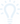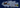# CALCULATE OEE

THE BIG IDEAUsing the preferred OEE calculation (A x P x Q = OEE) makes it easier to take action against the underlying causes of lost productivity.

## SIMPLE CALCULATION

The simplest way to calculate OEE is as the ratio of Fully Productive Time to Planned Production Time. Fully Productive Time is just another way of saying manufacturing only Good Parts as fast as possible (Ideal Cycle Time) with no Stop Time. Hence the calculation is:

OEE = (Good Count × Ideal Cycle Time) / Planned Production Time

Although this is an entirely valid calculation of OEE, it does not provide information about the three loss-related factors: Availability, Performance, and Quality. For that – we use the preferred calculation.

## PREFERRED CALCULATION

The preferred OEE calculation is based on the three OEE Factors: Availability, Performance, and Quality.OEE is calculated by multiplying the three OEE factors: Availability, Performance, and Quality.

### Availability

Availability takes into account all events that stop planned production long enough where it makes sense to track a reason for being down (typically several minutes).

Availability is calculated as the ratio of Run Time to Planned Production Time:

Availability = Run Time / Planned Production Time

Run Time is simply Planned Production Time less Stop Time, where Stop Time is defined as all time where the manufacturing process was intended to be running but was not due to Unplanned Stops (e.g., Breakdowns) or Planned Stops (e.g., Changeovers).

Run Time = Planned Production Time − Stop Time

### Performance

Performance takes into account anything that causes the manufacturing process to run at less than the maximum possible speed when it is running (including both Slow Cycles and Small Stops).

Performance is the ratio of Net Run Time to Run Time. It is calculated as:

Performance = (Ideal Cycle Time × Total Count) / Run Time

Ideal Cycle Time is the fastest cycle time that your process can achieve in optimal circumstances. Therefore, when it is multiplied by Total Count the result is Net Run Time (the fastest possible time to manufacture the parts).

Since rate is the reciprocal of time, Performance can also be calculated as:

Performance = (Total Count / Run Time) / Ideal Run Rate

Performance should never be greater than 100%. If it is, that usually indicates that Ideal Cycle Time is set incorrectly (it is too high).

### Quality

Quality takes into account manufactured parts that do not meet quality standards, including parts that need rework. Remember, OEE Quality is similar to First Pass Yield, in that it defines Good Parts as parts that successfully pass through the manufacturing process the first time without needing any rework.

Quality is calculated as:

Quality = Good Count / Total Count

This is the same as taking the ratio of Fully Productive Time (only Good Parts manufactured as fast as possible with no Stop Time) to Net Run Time (all parts manufactured as fast as possible with no stop time).

### OEE

OEE takes into account all losses, resulting in a measure of truly productive manufacturing time. It is calculated as:

OEE = Availability × Performance × Quality

If the equations for Availability, Performance, and Quality are substituted in the above and reduced to their simplest terms the result is:

OEE = (Good Count × Ideal Cycle Time) / Planned Production Time

This is the “simplest” OEE calculation described earlier. And, as described earlier, multiplying Good Count by Ideal Cycle Time results in Fully Productive Time (manufacturing only Good Parts, as fast as possible, with no Stop Time).

### Why the Preferred OEE Calculation?

OEE scores provide a very valuable insight – an accurate picture of how effectively your manufacturing process is running. And, it makes it easy to track improvements in that process over time.

What your OEE score doesn’t provide is any insights as to the underlying causes of lost productivity. This is the role of Availability, Performance, and Quality.

In the preferred calculation you get the best of both worlds. A single number that captures how well you are doing (OEE) and three numbers that capture the fundamental nature of your losses (Availability, Performance, and Quality).

Here is an interesting example. Look at the following OEE data for two sequential weeks.

OEE FactorWeek 1Week 2
OEE85.1%85.7%
Availability90.0%95.0%
Performance95.0%95.0%
Quality99.5%95.0%

OEE is improving. Great job! Or is it? Dig a little deeper and the picture is less clear. Most companies would not want to increase Availability by 5.0% at the expense of decreasing Quality by 4.5%.## CALCULATION EXAMPLE

Now let’s work through a complete example using the preferred OEE calculation. Here is data recorded for the first shift:

ItemData
Shift Length8 hours (480 minutes)
Breaks(2) 15 minute and (1) 30 minute
Downtime47 minutes
Ideal Cycle Time1.0 seconds
Total Count19,271 widgets
Reject Count 423 widgets

### Planned Production Time

As described in the OEE Factors page, the OEE calculation begins with Planned Production Time. So first, exclude any Shift Time where there is no intention of running production (typically Breaks).

Formula: Shift Length − Breaks

Example: 480 minutes − 60 minutes = 420 minutes

### Run Time

The next step is to calculate the amount of time that production was actually running (was not stopped). Remember that Stop Time should include both Unplanned Stops (e.g., Breakdowns) or Planned Stops (e.g., Changeovers). Both provide opportunities for improvement.

Formula: Planned Production Time − Stop Time

Example: 420 minutes − 47 minutes = 373 minutes

### Good Count

If you do not directly track Good Count, it also needs to be calculated.

Formula: Total Count − Reject Count

Example: 19,271 widgets − 423 widgets = 18,848 widgets

### Availability

Availability is the first of the three OEE factors to be calculated. It accounts for when the process is not running (both Unplanned Stops and Planned Stops).

Formula:Run Time / Planned Production Time

Example: 373 minutes / 420 minutes = 0.8881 (88.81%)

### Performance

Performance is the second of the three OEE factors to be calculated. It accounts for when the process is running slower than its theoretical top speed (both Small Stops and Slow Cycles).

Formula: (Ideal Cycle Time × Total Count) / Run Time

Example: (1.0 seconds × 19,271 widgets) / (373 minutes × 60 seconds) = 0.8611 (86.11%)

Performance can also be calculated based on Ideal Run Rate. The equivalent Ideal Run Rate in our example is 60 parts per minute.

Formula: (Total Count / Run Time) / Ideal Run Rate

Example: (19,271 widgets / 373 minutes) / 60 parts per minute = 0.8611 (86.11%)

### Quality

Quality is the third of the three OEE factors to be calculated. It accounts for manufactured parts that do not meet quality standards.

Formula: Good Count / Total Count

Example: 18,848 widgets / 19,271 widgets = 0.9780 (97.80%)

### OEE

Finally, OEE is calculated by multiplying the three OEE factors.

Formula: Availability × Performance × Quality

Example: 0.8881 × 0.8611 × 0.9780 = 0.7479 (74.79%)

OEE can also be calculated using the simple calculation.

Formula: (Good Count × Ideal Cycle Time) / Planned Production Time

Example: (18,848 widgets × 1.0 seconds) / (420 minutes × 60 seconds) = 0.7479 (74.79%)

The result is the same in both cases. The OEE for this shift is 74.79%.